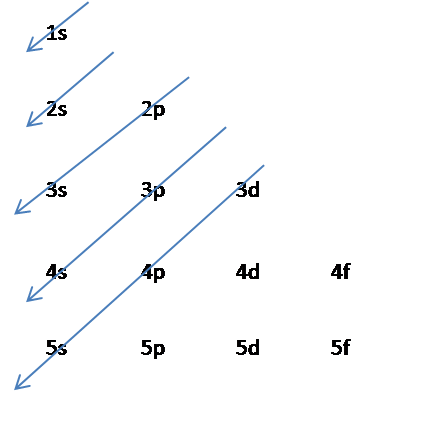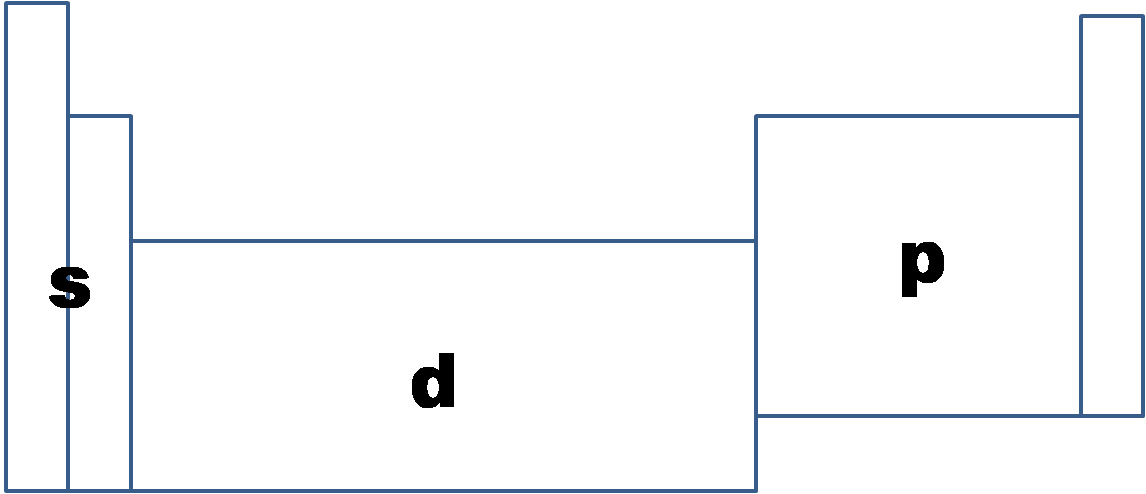# 1.9A: Ground State Electronic Configurations

$$\newcommand{\vecs}{\overset { \rightharpoonup} {\mathbf{#1}} }$$ $$\newcommand{\vecd}{\overset{-\!-\!\rightharpoonup}{\vphantom{a}\smash {#1}}}$$$$\newcommand{\id}{\mathrm{id}}$$ $$\newcommand{\Span}{\mathrm{span}}$$ $$\newcommand{\kernel}{\mathrm{null}\,}$$ $$\newcommand{\range}{\mathrm{range}\,}$$ $$\newcommand{\RealPart}{\mathrm{Re}}$$ $$\newcommand{\ImaginaryPart}{\mathrm{Im}}$$ $$\newcommand{\Argument}{\mathrm{Arg}}$$ $$\newcommand{\norm}{\| #1 \|}$$ $$\newcommand{\inner}{\langle #1, #2 \rangle}$$ $$\newcommand{\Span}{\mathrm{span}}$$ $$\newcommand{\id}{\mathrm{id}}$$ $$\newcommand{\Span}{\mathrm{span}}$$ $$\newcommand{\kernel}{\mathrm{null}\,}$$ $$\newcommand{\range}{\mathrm{range}\,}$$ $$\newcommand{\RealPart}{\mathrm{Re}}$$ $$\newcommand{\ImaginaryPart}{\mathrm{Im}}$$ $$\newcommand{\Argument}{\mathrm{Arg}}$$ $$\newcommand{\norm}{\| #1 \|}$$ $$\newcommand{\inner}{\langle #1, #2 \rangle}$$ $$\newcommand{\Span}{\mathrm{span}}$$$$\newcommand{\AA}{\unicode[.8,0]{x212B}}$$

Ground state electron configurations are the foundation for understanding molecular bonding, properties, and structures. From the electrons in an atom, to the differing orbitals and hybridization, the ground state electron configuration sheds light on many different atomic properties. Fundamentally, understanding electron configuration leads to an understanding of the periodic table.

## Introduction

In 1913, Niels Bohr proposed that electrons could orbit an atom at a certain distance without collapsing into the atom, and that each orbit distance had its own energy level. He proposed that each orbital’s angular momentum, M, was equal to a multiple, n, of Plank’s constant, h, divided by 2π. This gives the equation:

M = nħ where ħ= h/2π and n= 1,2,3,4

This model proposed the Bohr atom, which shows circular orbits surrounding the nucleus.### Orbitals

In addition to having different energy levels, orbitals also have different shapes and orientations, and each can be occupied by two electrons. For each principal quantum number, n, there is one s orbital, three p orbitals, five d orbitals and seven f orbitals. Therefore, an s orbital can hold two electrons, a p orbital can hold six electrons, a d orbital can hold ten electrons, and an f orbital can hold 14 electrons.

## Ground State Electron Configuration

### Quantum numbers

There are four quantum numbers n, l, ml, and ms. The principal quantum number n is a positive integer (1,2,3,4) and it represents the energy of the orbital. The angular momentum quantum number l, is from 0 to n – 1. The l values of 0, 1, 2, and 3 correspond to the s, p, d and f orbitals, respectively. The magnetic quantum number ml ranges from –l to +l. This quantum number dictates the orbital orientation, such as px, py, or pz. The quantum spin number ms, is either +1/2 or -1/2 and it dictates the electron spin.

### Aufbau Principle

The Aufbau principle states that electrons must fill lowest energy shells first.Following the model, electrons fill the 1s orbital with two electrons, then the 2s with two electrons, then the 2p with six electrons, then the 3s with two electrons, etc.

There are some exceptions to the Aufbau Principle. This occurs mainly with electrons in the d orbital where extra stability is obtained from a half filled or fully filled d orbital. Therefore, if there are 4 electrons, or 9 electrons in the d orbital, it will move one electron from the s orbital below it to fill the extra space.

##### Example $$\PageIndex{1}$$: Chromium

The electron configuration of chromium

###### Solution

Cr's electron configuration, following the model would be: $$1s^2 2s^2 2p^6 3s^2 3p^6 4s^23d^4\0, but instead it is \(1s^2 2s^2 2p^6 3s^2 3p^6 4s^13d^5$$, because there is extra stability gained from the half-filled d orbital.

### Hund's Rule

Hund’s rule states that when filled sub-levels other than s orbital, electrons must not be spin paired in the orbitals until each orbital contains one electron, and no orbital can have two electrons with the same spin (ms).

### Pauli Exclusion Principle

Pauli Exclusion Principle states that no two electrons can have the same quantum numbers. An orbital can only hold 0, 1, or 2 electrons. They must have opposite spins if there are 2 electrons in the orbittal.

### Periodic Trend

Valence electron shells in the periodic table follow a trend. This can be referred to as the s block, the p block, the d block and the f block (lanthanides and actinides) meaning that, in its ground state, an element in a certain "block" will have its valence electrons in the s, p, d, or f orbitals depending.## How to Write Ground State Electron Configurations

### Basics

Electron configurations are written using the principal quantum number n, followed by the orbital (s, p, d, or f) with the total number of electrons written as a superscript. Example: 1s2 For writing ground state electron configurations, a few main steps should be followed.

1. Find the amount of electrons in the atom. Example: Na: 11 e- Na+: 10 e-
2. Fill orbitals following the model until all electrons have been accounted for.Example: Na: 11 e- 1s2 2s2 2p6 3s1 or Na+: 1s2 2s2 2p6

1. After that, it is important to check for a nearly half-filled or filled d orbital (d4 or d9) and adjust accordingly by removing an electron from the s orbital beneath it.
##### Example $$\PageIndex{2}$$: Chromium

What is the electronic configuration of chromium

###### Solution

Cr: 1s2 2s2 2p6 3s2 3p64s23d4 half filled orbital, s orbital beneath it

1s2 2s2 2p6 3s2 3p6 4s13d5

### Shorthand

Because writing the entire electron configuration can become cumbersome, there is a shorthand option. It is done by using the symbol of the noble gas in the period above the element to represent the electron configuration before it.

Example: Na: [Ne] 3s1

## References

1. Housecroft, Catherine E. and Alan G. Sharpe, “Inorganic Chemistry,” 3rd ed. England: Pearson Education Limited: 2008.
2. Tro, Nivaldo J. “Chemistry: A Molecular Approach,” 8th ed. Upper Saddle River, New Jersey: Prentice Hall: 2007.
3. Silberberg, Martin S. "Chemistry: The Molecular Nature of Matter and Change," 4th ed. Boston: McGraw Hill: 2006.